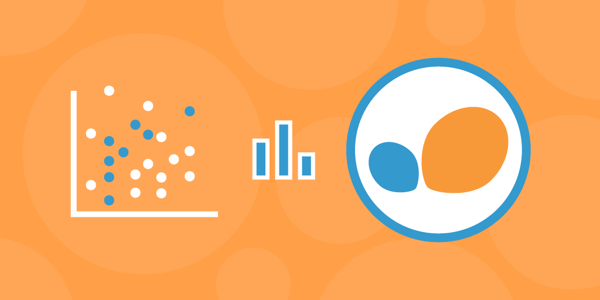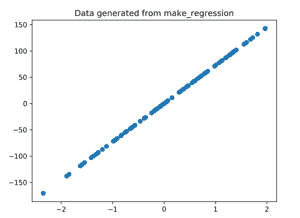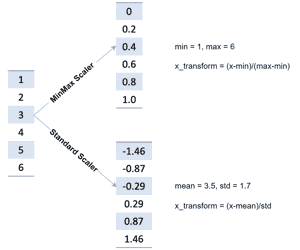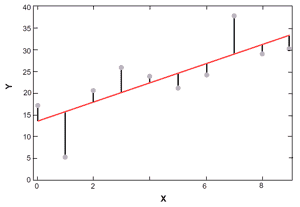# Scikit-learn tutorial: How to implement linear regression

Oct 13, 2020 - 11 min readRyan ThelinMachine learning is quickly becoming the most sought after skill in the job market. Most employers are specifically looking for candidates with experience in Scikit-learn, the most popular ML Python library. Scikit-learn is a library for Python that provides machine learning developers with many unsupervised and supervised learning algorithms.

Today, we’ll explore this awesome library and show you how to implement its core functions. In the end, we’ll combine what we’ve learned to implement your own linear regression algorithm.

Here’s what we’ll cover today:

## What is Scikit-Learn?

Scikit-learn (or sklearn for short) is a free open-source machine learning library for Python. It is designed to cooperate with SciPy and NumPy libraries and simplifies data science techniques in Python with built-in support for popular classification, regression, and clustering machine learning algorithms.

Sklearn serves as a unifying point for many ML tools to work seamlessly together. It also gives data scientists a one-stop-shop toolkit to import, preprocess, plot, and predict data.

The project was started by David Cournapeau during the 2007 Google Summer of Code, and this library has grown over the last decade in both popularity and features. Scikit-learn is now the most popular machine learning library on Github.

Scikit-learn provides tools for:

• Regression, including Linear and Logistic Regression
• Classification, including K-Nearest Neighbors
• Model selection
• Clustering, including K-Means and K-Means++
• Preprocessing, including Min-Max Normalization

Developers and machine learning engineers use Sklearn because:

• It’s easy to learn and use.
• It’s free and open-source.
• It helps in all aspects and algorithms of Machine Learning, even Deep Learning.
• It’s very versatile and powerful.
• Detailed documentation and active community.
• It is the most widely used Machine Learning toolkit.

### Libraries used with Scikit-learn

Scikit-learn is a tool kit to expand the functions of the existing SciPy Stack (sometimes called the NumPy Stack). Below, we outline how Scikit-learn uses each library within the SciPy stack for data analysis.

• NumPy: Advanced linear algebra and NumPy array operations
• SciPy: Contains modules for optimization, linear algebra, and other essential data science functions.
• Matplotlib: Visualization and data plotting in 2 or 3 dimensions.
• IPython: Increasing console interactivity.
• SymPy: Symbolic computation and computer algebra.
• Pandas: Data manipulation and analysis, mainly through dataframes and tables.

## Getting started with Scikit-learn

Today, I’ll show you how to implement your own linear regression algorithm with scikit learn. Before we begin, you’ll need some foundational knowledge of:

• The purpose of ML and data science.
• How machine learning algorithms differ from one another.
• Linear algebra, how it relates to ML.

### Import Scikit-learn

First, you’ll need to install Scikit-Learn. We’ll use pip for this, but you may also use conda if you prefer.

For Scikit-learn to work correctly, you’ll need a 64-bit version of Python 3, and the NumPy and SciPy libraries. For visual data plots, you’ll also need matplotlib.

To install Scikit-learn enter the following line into your Python 3.

 pip install -U scikit-learn


Then to verify the installation, enter:

python -m pip show scikit-learn # displays which version and where sklearn is installed
python -m pip freeze # displays all packages installed in virtualenv
python -c "import sklearn; sklearn.show_versions()"


Linux users: add 3 after pip and python in the above lines → pip3, python3.

Now to install NumPy, SciPy and, matplotlib, enter:

pip install -U numpy
pip install -U scipy
pip install -U matplotlib


As we did before, we’ll confirm the installation of each with:

python -m pip show numpy
python -m pip show scipy
python -m pip show matplotlib


Now you’re ready to start using Scikit-learn! Let’s jump into our tutorial by importing a dataset.

## Datasets and import.sklearn

The starting point for all machine learning projects is to import your dataset. Scikit-learn includes three helpful options to get data to practice with.

First, the library contains famous datasets like the iris classification dataset or the Boston housing price regression set if you want to practice on a classic set. You can also use Scikit-learn’s predefined functions to download real-world datasets directly from the internet, such as 20 newsgroups.

Finally, you can simply generate a random dataset to match a certain pattern using Scikit-learn’s data generator. Each of these options requires you to import the datasets module:

import sklearn.datasets as datasets


First, we’ll import the iris classification set to see how it’s stored in sklearn.

iris = datasets.load_iris()


The iris data set is imported as a dictionary-like object with all necessary data and metadata. The data is stored in the 2D array data field of n_samples * n_features.

We can get descriptions of the data and its formatting by using the DESCR, shape, and _names functions. If we print the results of these functions we’ll discover all the information we could need to work with the iris set.

Targets and Features:

All ML algorithms attempt to increase their understanding of a certain variable, called the target variable. The algorithm then attempts to uncover an unseen relationship between the target variable and other passed feature variables.

import sklearn.datasets as datasets

print("iris dataset is {}".format(iris.DESCR))
print("iris data size is {}".format(iris.data.shape))
print("iris target size is {}".format(iris.target.shape))
print("iris data has {} features, the feature names are {}".format(
iris.data.shape, iris.feature_names))
print("iris data has {} samples, the target label names {}".format(
iris.data.shape, iris.target_names))

## Generate synthetic regression data

If you don’t want to use any of the built-in datasets, you can generate your own data to match a chosen distribution. Below, we’ll see how to generate regression data and plot it using matplotlib.

First, import matplotlib using:

import matplotlib.pyplot as plt


Now, we’ll generate a simple regression data set with 1 feature and 1 informative feature.

X, y = datasets.make_regression(n_features=1, n_informative=1)


This generates our dataset and saves it to 2D dataset object x, y. Changing the parameters of the make_regression function will alter the characteristics of the data generated. Here, we change the features and informative parameters from their default 10 to instead be just 1.

Other popular parameters include samples that control the number of samples and targets that control how many target variables are tracked.

Informative vs non-informative feature:

An informative feature is one that provides useful, applicable information to the ML algorithm. These are the data points used to form the trend in regression analysis algorithms. Non-informative features are discarded as unhelpful.

### Plotting data with matplotlib

We’ll now plot this graph by entering:

fig, axe = plt.subplots(dpi = 300)
axe.scatter(X, y, marker='o')
axe.set_title("Data generated from make_regression")
fig.savefig("output/img.png")
plt.close(fig)

• Line 1 unpacks the plot tuple variable into separate variables fig and axe. This allows us to save the figure and manipulate these separate attributes.

• Line 2 generates a scatter plot using our generated dataset object x,y. The marker parameter determines what visual will be used to mark each data point, in this case, a dot (o).

• Line 3 sets the title of our generated plot.

• Line 4 and 5 then save the figure to a .png image file and close the current figure.Generated regression plot

Master all the top ML algorithms you’ll need to pass an ML interview. Educative’s text-based courses are designed by industry experts to teach you the most sought after Scikit-learn techniques out there.

Hands-on Machine Learning with Scikit-Learn

## Data Preprocessing

Most ML engineers agree that data preprocessing is one of the most important steps in the project process. No dataset is perfect: there can be an extraneous data point, reporting errors, and any number of issues that interfere with an algorithm’s prediction.

To prevent this, data scientists spend many hours cleaning, normalizing, and scaling data long before it ever passes into an ML algorithm.

The most common function type you’ll use in this stage are standardizing functions, namely the MinMax and Standard functions. This is because features in your data will vary in range. However, nearly all ML algorithms use Euclidean distance to measure the distance between two data points.

Scale standardization functions allow algorithms to properly measure distance by scaling all points in the set to fit the same range.

Both will require you to first import sklearn.preprocessing and numpy:

import sklearn.preprocessing as preprocessing
import numpy as np


### MinMax

MinMax shrinks the range of each figure to be between 0 and 1.

​​
import sklearn.preprocessing as preprocessing

minmax = preprocessing.MinMaxScaler()
## X is a matrix with float type
minmax.fit(X)
X_minmax = minmax.transform(X)

• Line 3 creates a MinMaxScaler named minmax.
• Line 5 fits the original scale matrix to the Scaler
• Line 6 transforms the original matrix to match the fitted matrix X

Here’s an example of our MinMaxScalar in action!

import sklearn.preprocessing as preprocessing
import numpy as np

X = np.random.randint(2, 10, size=(4, 2))
X2 = np.random.randint(100, 10000, size=(4, 2))
X = np.concatenate((X, X2), axis=1)
print("The original matrix")
print(X)

#### min-max scaler

minmax = preprocessing.MinMaxScaler()
minmax.fit(X)
X_minmax = minmax.transform(X)
print("The transform data using min-max scaler")
print(X_minmax)

​​At first, we use Numpy randint to create a matrix with a size of four rows and two columns, whose number ranges from two to ten.

Then, we create another matrix with the same size, whose numbers range from 100 to 1,000. These two metrics are concatenated into one.

From the output of line 8, you can see that the range of numbers varies greatly for different columns. We then apply our min-max scaler to shrink and standardize the range between features.

### Standard

If your data instead follows standard deviation, you can use the StandardScaler instead. This scaler fits a passed data set to be a standard scale along with the standard deviation.

import sklearn.preprocessing as preprocessing

std = preprocessing.StandardScaler()
## X is a matrix
std.fit(X)
X_std = std.transform(X)


Like above, we first create the scaler on line 3, fit the current matrix on line 5, and finally transform the original matrix on line 6.

Let’s see how this scales our same example from above:

import sklearn.preprocessing as preprocessing
import numpy as np

X = np.random.randint(2, 10, size=(4, 2))
X2 = np.random.randint(100, 10000, size=(4, 2))
X = np.concatenate((X, X2), axis=1)
print("The original matrix")
print(X)

std = preprocessing.StandardScaler()
std.fit(X)
X_std = std.transform(X)
print("The transform data using Standard scaler")
print(X_std)Scaling method comparison

### Scikit-learn Linear Regression: implement an algorithm

Now we’ll implement the linear regression machine learning algorithm using the Boston housing price sample data. As with all ML algorithms, we’ll start with importing our dataset and then train our algorithm using historical data.

Linear regression is a predictive model often used by real businesses. Linear regression seeks to predict the relationship between a scalar response and related explanatory variables to output value with realistic meaning like product sales or housing prices.

This model is best used when you have a log of previous, consistent data and want to predict what will happen next if the pattern continues.

From a mathematical point of view, linear regression is about fitting data to minimize the sum of residuals between each data point and the predicted value. In other words, we are minimizing the discrepancy between the data and the estimation model.

As shown in the figure below, the red line is the model we solved, the blue point is the original data, and the distance between the point and the red line is the residual. Our goal is to minimize the sum of residuals.Sum of residuals, data vs prediction

#### How to implement linear regression

import sklearn.datasets as datasets
from sklearn.model_selection import train_test_split
from sklearn.linear_model import LinearRegression
import sklearn.metrics as metrics

print("The data shape of house is {}".format(house.data.shape))
print("The number of feature in this data set is {}".format(
house.data.shape))

train_x, test_x, train_y, test_y = train_test_split(house.data,
house.target,
test_size=0.2,
random_state=42)

print("The first five samples {}".format(train_x[:5]))
print("The first five targets {}".format(train_y[:5]))
print("The number of samples in train set is {}".format(train_x.shape))
print("The number of samples in test set is {}".format(test_x.shape))

lr = LinearRegression()
lr.fit(train_x, train_y)

pred_y = lr.predict(test_x)
print("The first five prediction {}".format(pred_y[:5]))
print("The real first five labels {}".format(test_y[:5]))

mse = metrics.mean_squared_error(test_y, pred_y)
print("Mean Squared Error {}".format(mse))

• At line 6, we load the dataset by calling load_boston.

• At line 12, we split the dataset into two parts: the train set (80%), and the test set (20%).

• At line 23, A linear regression model is created and trained at (in sklearn, the train is equal to fit).

• At line 29, we call mean_squared_error to evaluate the performance of this model.

### Wrapping up and next steps

You’ve just taken your first steps to master Scikit-Learn. Today, we covered the purpose of Sklearn, how to import or generate sample data, how to scale our data, and how to implement the popular linear regression algorithm.

As you continue your Scikit-learn journey, here are some next algorithms and topics to learn:

• Support Vector machine
• Random Forest
• Naive Bayes model
• Unsupervised learning
• Deep learning
• Logistic regression

To help you get started, Educative has created the course Hands-on Machine Learning with Scikit-Learn. With in-depth explanations of all the Scikit-learn basics and popular ML algorithms, this course will give you everything you need in one place. By the end of this course, you’ll know how and when to use each algorithm and will have the Scikit skills to stand out to any interviewer.